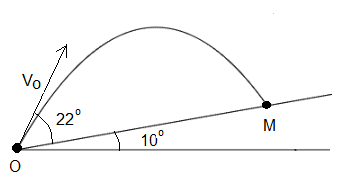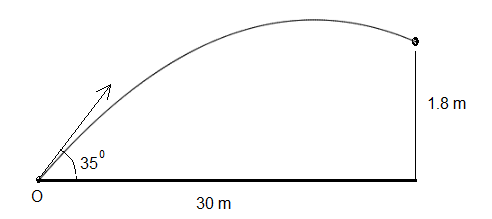# Projectile Problems with Solutions and Explanations

Projectile problems are presented along with detailed solutions. These problems may be better understood when projectile equations are first reviewed. An interactive html 5 applet may be used to better understand the projectile equations.

## Problems with Detailed Solutions

### Problem 1

An object is launched at a velocity of 20 m/s in a direction making an angle of 25� upward with the horizontal.
a) What is the maximum height reached by the object?
b) What is the total flight time (between launch and touching the ground) of the object?
c) What is the horizontal range (maximum x above ground) of the object?
d) What is the magnitude of the velocity of the object just before it hits the ground?
Solution to Problem 1

### Problem 2

A projectile is launched from point O at an angle of 22� with an initial velocity of 15 m/s up an incline plane that makes an angle of 10� with the horizontal. The projectile hits the incline plane at point M.
a) Find the time it takes for the projectile to hit the incline plane.
b)Find the distance OM.Solution to Problem 2

### Problem 3

A projectile is to be launched at an angle of 30� so that it falls beyond the pond of length 20 meters as shown in the figure.
a) What is the range of values of the initial velocity so that the projectile falls between points M and N?Solution to Problem 3

### Problem 4

A ball is kicked at an angle of 35� with the ground.
a) What should be the initial velocity of the ball so that it hits a target that is 30 meters away at a height of 1.8 meters?
b) What is the time for the ball to reach the target?Solution to Problem 4

### Problem 5

A ball kicked from ground level at an initial velocity of 60 m/s and an angle θ with ground reaches a horizontal distance of 200 meters.
a) What is the size of angle θ?
b) What is time of flight of the ball?
Solution to Problem 5

### Problem 6

A ball of 600 grams is kicked at an angle of 35� with the ground with an initial velocity V0.
a) What is the initial velocity V
0 of the ball if its kinetic energy is 22 Joules when its height is maximum?
b) What is the maximum height reached by the ball
Solution to Problem 6

### Problem 7

A projectile starting from ground hits a target on the ground located at a distance of 1000 meters after 40 seconds.
a) What is the size of the angle θ?
b) At what initial velocity was the projectile launched?
Solution to Problem 7

### Problem 8

The trajectory of a projectile launched from ground is given by the equation y = -0.025 x2 + 0.5 x, where x and y are the coordinate of the projectile on a rectangular system of axes.
a) Find the initial velocity and the angle at which the projectile is launched.
Solution to Problem 8

### Problem 9

Two balls A and B of masses 100 grams and 300 grams respectively are pushed horizontally from a table of height 3 meters. Ball has is pushed so that its initial velocity is 10 m/s and ball B is pushed so that its initial velocity is 15 m/s.
a) Find the time it takes each ball to hit the ground.
b) What is the difference in the distance between the points of impact of the two balls on the ground?
Solution to Problem 9